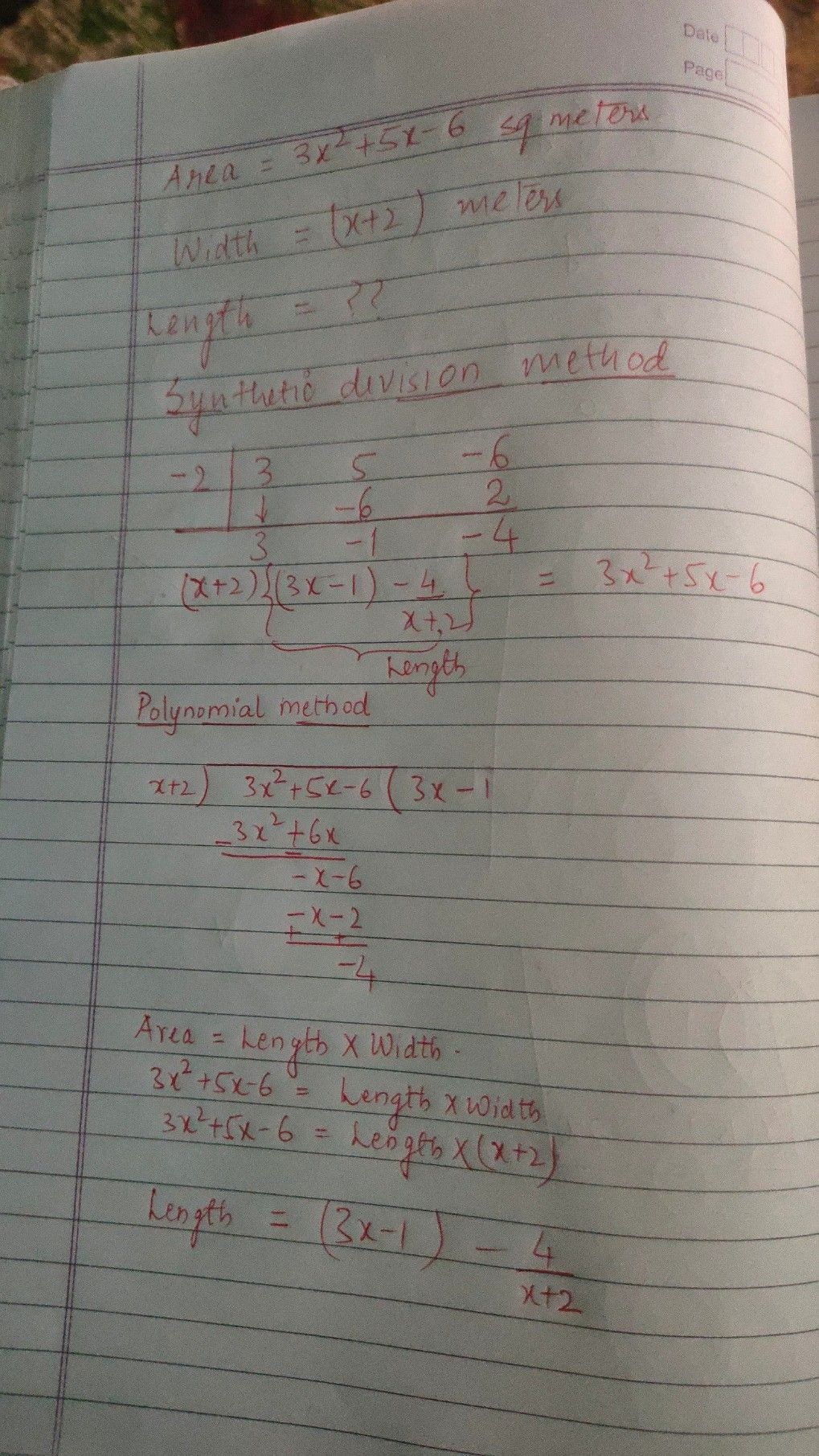Symbol
ProblemHere is another activity that lets you apply what $yo11$ learned about the division of polynomials using long division and synthetic division by relating it to $ea1-lif_{0}$ situations. Solve, then Decide Solve the problem. Show your complete solution - $P$ A rectangular $gardcn$ $ina$ backyard $has$ $an$ area $of\left(3x^{2}+5x-6\right)$ square meters. $1ts$ width is $\left(x+2\right)$ meter a. Find the length of the garden. b. You decided to partition the garden $int0$ two or more smaller congruent gardens. Design $a$ $possib16$ model and include mathematical concept in your design